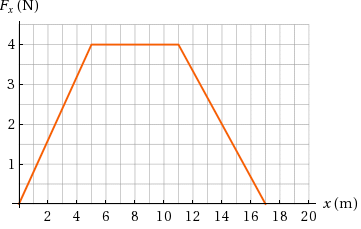Question
15 views

A object of mass 3.00 kg is subject to a force Fx that varies with position as in the figure below.

If the object has a speed of 0.400 m/s at x=0

find its speed at x=5.00 m

and its speed at x=17.0 mhelp_outlineImage TranscriptioncloseF, (N) 4 1 x (m) 10 12 14 16 18 20 2 4 6 8 3. 2. fullscreen
check_circle

Step 1

Area under force-displacement graph will give the work done.

Area under graph from x=0 m to x=5 m is,

Step 2

According to work energy theorem, work done will equal to change in kinetic energy.

Step 3

Area under graph from x=0 m to ...

### Want to see the full answer?

See Solution

#### Want to see this answer and more?

Solutions are written by subject experts who are available 24/7. Questions are typically answered within 1 hour.*

See Solution
*Response times may vary by subject and question.
Tagged in

### Physics